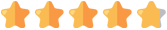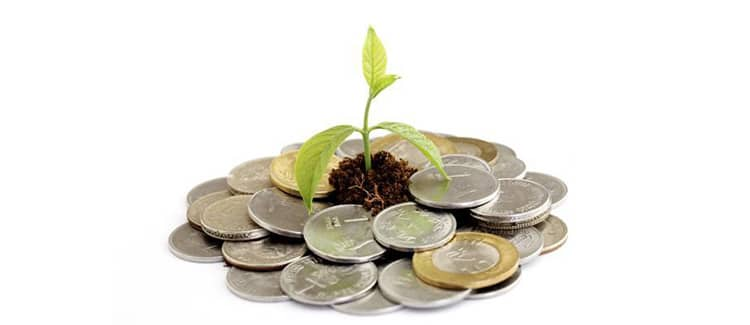# HDFC Bank Fixed Deposit (FD) Interest Calculator

The HDFC Bank FD calculator is an online tool that helps a person to calculate the maturity amount for a fixed deposit in HDFC Bank. It helps an applicant to determine the returns that he/ she will earn on investing an amount in an HDFC Bank FD for a certain period of time. This calculator uses information such as the FD investment amount, tenure, applicable HDFC Bank FD rates and frequency of interest calculation.

Best Investment Options
• Save upto ₹46,800 in tax under Sec 80C

• Inbuilt Life Cover

• Tax Free Returns Unlike FD+

*All savings are provided by the insurer as per the IRDAI approved insurance plan. Standard T&C Apply
+ Trad plans with a premium above 5 lakhs would be taxed as per applicable tax slabs post 31st march 2023
~Source - Google Review Rating available on:- http://bit.ly/3J20bXZ

Get Guaranteed returns along with life cover
invest in 100% Guaranteed Return Plans Tax benefits under sec 80C & No Tax on returns+
+91We don’t spam
View Plans
Plans available only for people of Indian origin By clicking on ''View Plans'' you, agreed to our Privacy Policy and Terms of use #For a 55 year on investment of 20Lacs #Discount offered by insurance company Tax benefit is subject to changes in tax laws
We are rated~6.7 Crore
Registered Consumers
51
Insurance Partners
3.4 Crore
Policies Sold
HDFC Bank FD Calculator

Total Investment

₹500 ₹30L
Enter Total Investment

Rate of Interest (Yearly)

1% 15%
Rate of Interest (Yearly)

Time Period

1 Year 15 Years
Enter Time PeriodTotal InvestmentInterest EarnedMaturity Amount

Fixed deposit is a great way to invest in a risk-free financial instrument and plan a better future. The HDFC Bank allows a person to park his/ her savings in an FD and earn handsome returns on it until maturity. The interest on an HDFC Bank FD can be earned every month, every quarter, every six months or once a year. In case senior citizens apply for a fixed deposit in HDFC Bank, they will earn 0.50% higher interest on FD rates applicable to the general public.

## How Can HDFC Bank Fixed Deposit Calculator Help?

The HDFC Bank provides different types of fixed deposit schemes to its customers. Each type of FD earns an attractive interest on the investment amount at a rate depending on the FD tenure. A fixed deposit in HDFC Bank is available for a tenure of at least 7 days and a maximum of 10 years. A person should opt for a mix of short-term and long-term fixed deposits for better financial planning. An HDFC FD calculator can help in the following ways:

• Determine the Maturity Amount - An HDFC fixed deposit calculator helps a person to estimate the maturity amount before applying for an FD. This allows the investor to determine which FD scheme he/ she should invest in to earn maximum returns.

• Helps to Choose FD Tenure - It helps to determine how much returns can be earned on an investment amount with respect to different tenures. As a result, an investor can decide which tenure can help them fulfil their investment goals.

• Provides More Accuracy - It provides greater accuracy when it comes to calculating the maturity amount as compared to manual calculations. Thus, an HDFC FD interest rates calculator is efficient and provides accurate results.

• Plan Financial Investments - An HDFC FD interest calculator helps a person to plan his/ her financial investments. They can use the tool to find out which FD scheme and tenure brings them the closest to their short-term/ long-term investment goals.

• Saves Time & Resources - It saves a lot of time as well as money by providing the end result of an HDFC Bank FD investment instantly.## How Does an HDFC Bank FD Calculator Work?

An HDFC FD rates calculator work by using a formula to calculate the maturity amount for a fixed deposit. The applicant needs to provide the details of fixed deposit, such as the FD amount, tenure, interest rate applicable and the interest calculation frequency for the calculator to determine its maturity amount. Take a look at the formula used by an HDFC FD calculator:

A= P(1+r/n)^n*t

In the above formula,

‘A’ stands for the fixed deposit maturity amount

‘P’ stands for the amount you invest in the FD

‘r’ stands for the HDFC Bank FD rates

‘n’ stands for the number of times interest on an FD is calculated. ‘N’ can equal to ‘1’ if the interest is calculated annually, ‘2’ if calculated half-yearly, ‘4’ if calculated quarterly and ‘12’ if calculated every month

‘t’ stands for the tenure of the fixed deposit

Let’s understand the formula with the help of an example:

Suppose a person wants to apply for an FD of Rs 1.2 lakh in HDFC Bank for a period of 8 years and the applicable interest rate is of 6.5% which is calculated every quarter. In this case, an HDFC fixed deposit calculator will apply the given information on the above-mentioned formula in the following way:

P = 1,20,000

r = 6.5%

n = 4

t = 8 years

Thus, A = 1,20,000 (1+6.5/4)^4*8 which comes out to Rs 2,01,001.

Therefore, the depositor will get a maturity amount of Rs 2,01,001 after 8 years.

## Simple Interest vs. Compound Interest

The HDFC Bank calculates the interest on a fixed deposit using two methods, i.e. simple interest and compound interest method. Here are some of the major difference between simple interest and compound interest method of calculating FD interest:

 Categories Simple Interest on HDFC Bank FD Compound Interest on HDFC Bank FD Meaning Simple interest method calculates the interest on an HDFC Bank FD amount on a fixed rate of interest for a specified tenure. Compound interest method calculates the interest and adds it to the fixed deposit principal  amount. The interest is compounded and paid only at FD maturity. Investment/ Principal Amount Under the simple interest method, the interest is calculated on the investment amount or the principal amount with which the FD account was opened. Under the compound interest method, the interest is calculated on the principal amount and not on the original investment amount. The interest earned in the first interval (month/ year) is added to the original principal amount and their total becomes the principal amount for the next interval. The procedure continues until the FD matures. Change in Principal Amount The principal amount under this method remains unchanged until the FD matures. The principal amount changes at every interval (month/ quarter) as the interest is added to the principal amount and the sum of the two becomes the new principal amount. Returns Earned Lower returns are earned under simple interest method as the principal amount does not change throughout the FD tenure. Higher returns can be earned under the compound interest method as the principal amount increases at every interval or period. Formula The formula of simple interest is P*R*T/ 100. Under this formula, ‘P’ stands for the principal amount invested in an FD, ‘R’ stands for the HDFC Bank FD rates applicable and ‘T’ stands for the maturity period. The formula of compound interest is P (1+R/100) - P. In this formula, ‘P’ refers to the principal amount of the FD and ‘R’ refers to applicable HDFC Bank interest rate.

## How to Use PolicyBazaar HDFC Bank Fixed Deposit Calculator?

Anyone can easily use the HDFC Bank FD calculator available on policybazaar.com to calculate the maturity amount of their fixed deposit. Just follow the steps given below to use an HDFC fixed deposit calculator:

• Visit the website of policybazaar.com

• Go to the HDFC Bank FD calculator

• Fill in the amount that you wish to invest

• Enter the tenure of your FD along with the applicable HDFC Bank FD rates

• Choose if you are a senior citizen

• Click on ‘Calculate’ to submit the details

• The calculator will display the maturity amount of your FD

## Advantages of Using HDFC Bank Fixed Deposit Calculator

The HDFC FD calculator provides the following advantages to a fixed deposit applicant:

• It allows a person to know beforehand the maturity amount for a fixed deposit in HDFC Bank.

• It is easy to use, reliable and provides accurate results.

• It helps you to build your financial portfolio by helping you choose the FD schemes that will fulfil your investment goals.

• It helps a person to compare the returns on different HDFC Bank FD schemes as well as those available in other banks.

• The FD calculator tool can be used for free and at any time as it is available online.

## HDFC Fixed Deposit - FAQs

• ### Q.How can I open a fixed deposit in HDFC Bank?

Ans:. You can open a fixed deposit in HDFC Bank online by visiting its official website or mobile application. You can also visit your nearest HDFC Bank branch to open your FD offline.
• ### Q. What is the interest payment option under HDFC Bank FDs?

Ans:. The HDFC Bank provides two types of interest payment option under their fixed deposit schemes:
• Periodic Interest Payment - Under this option, the HDFC Bank pays the interest earned on the FD at each interval or period (such as quarterly, semi-annually) and credits it to your account.
• Cumulative Deposit - Under this option, the Bank credits the cumulative interest earned on the principal amount to your HDFC Bank account on maturity.
• ### Q. How many years will my HDFC Bank FD double in value?

Ans:. To know the number of years in which your fixed deposit amount in HDFC Bank will double up, you need to apply the rule of 72. As per the rule, divide the number 72 with the rate of interest applicable on your FD to know the number of years it will take for your fixed deposit to double up.

For instance, if a 5.5% HDFC Bank FD interest rate is applicable on your deposit, then divide 72/5.5, which will come to 13.09. Thus, it will take about 13 years for your HDFC Bank FD to double its value.

• ### Q. What is the maximum amount I can invest in an HDFC Bank FD?

Ans:. You can open an HDFC Bank fixed deposit with any amount of your choice as there is no maximum investment limit for an FD in this Bank.
• ### Q. How much amount do I need to open an FD in HDFC Bank?

Ans:. You need at least Rs 5,000 to open a fixed deposit in HDFC Bank.
• ### Q. Can I change the maturity period of my ongoing FD?

Ans:. No. You cannot change the maturity period of an ongoing FD in HDFC Bank.Disclaimer: Policybazaar does not endorse, rate or recommend any particular insurer or insurance product offered by an insurer.

### FD Rates articles

Recent Articles
Popular Articles31 Aug 2023

Banks in India play an important role in the country's financial14 Aug 2023

Karur Vysya Bank or KVB FD interest rates range from 4.00% to10 Jul 2023

एचडीएफसी एफडी ब्याज18 Apr 2023

Savings and emergency funds are two crucial aspects of financial12 Apr 2023

Banks offer the facility to avail of a loan against FixedFixed Deposits are the safest investment instruments. You invest the amount of your choice as the fixed depositThe monthly income scheme of Fixed Deposit has gained popularity among investors. It is considered liquid as itICICI offers various fixed deposit schemes which are highly liquid. The fixed deposit schemes allow partial